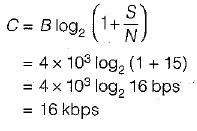Courses

# Information Theory & Coding

## 10 Questions MCQ Test Topicwise Question Bank for Electronics Engineering | Information Theory & Coding

Description
This mock test of Information Theory & Coding for GATE helps you for every GATE entrance exam. This contains 10 Multiple Choice Questions for GATE Information Theory & Coding (mcq) to study with solutions a complete question bank. The solved questions answers in this Information Theory & Coding quiz give you a good mix of easy questions and tough questions. GATE students definitely take this Information Theory & Coding exercise for a better result in the exam. You can find other Information Theory & Coding extra questions, long questions & short questions for GATE on EduRev as well by searching above.
QUESTION: 1

### The probabilities of the five possible outcomes of an experiment are given as: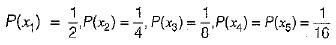If there are 16 outcomes per second then the rate of information would be equal to

Solution:

The entropy of the system is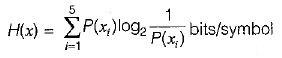or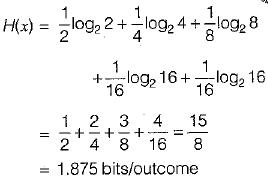Now, rate of outcome r= 16 outcomes/sec (Given)

∴ The rate of information R is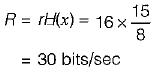QUESTION: 2

### Consider the binary Hamming code of block length 31 and rate equal to (26/31). Its minimum distance is

Solution:

Minimum distance in hamming code = 3.

QUESTION: 3

### Assertion (A): The Shannon-Hartley law shows that we can exchange increased bandwidth for decreased signal power for a system with given capacity C. Reason (R): The bandwidth and the signal power place a restriction upon the rate of information that can be transmitted by a channel.

Solution:

According to Shannon-Hartley faw, the channel capacity is expressed as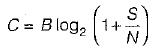Thus, if signal power is more, bandwidth will be less and vice-versa. Thus, assertion is a true statement. Reason is also a true statement because the rate of information that can be transmitted depends on bandwidth and signal to noise power. Thus, both assertion and reason are true but reason is not the correct explanation of assertion.

QUESTION: 4

A 1200 band data stream is to be sent over a non-redundant frequency hopping system, The maximum bandwidth for the spread spectrum signal is 10 MHz. if no overlap occurs, the number of channels are equal to

Solution: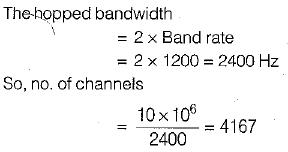QUESTION: 5

The differential entropy H(x) of the uniformly distributed random variable* with the following probability density function for a = 1 is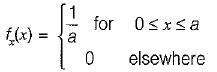Solution:

We know that the differential entropy of x is given by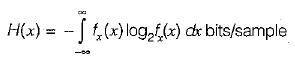Using the given probability density function, we have: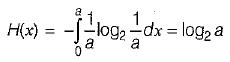for

a = 1, H(x) = log2 1 = 0

QUESTION: 6

During transmission over a communication channel bit errors occurs independently with probability 1/2 . If a block of 3 bits are transmitted the probability of at least one bit error is equal to

Solution:

(1 - p)n + np{ 1 - p)n-1 = Required probability
(Here, n = no. of bits and p = probability) ∴  Required probability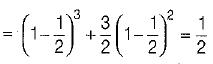QUESTION: 7

A channel has a bandwidth of 8 kHz and signal to noise ratio of 31. For same channel capacity, if the signal to noise ratio is increased to 61, then, the new channel bandwidth would be equal to

Solution: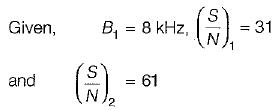We know that channel capacity is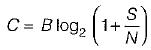Since channel capacity remains constant, therefore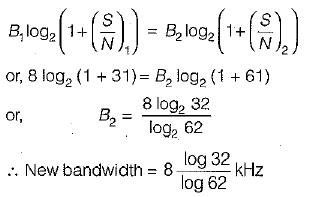QUESTION: 8

A source generates 4 messages. The entropy of the source will be maximum when

Solution:
QUESTION: 9

A source delivers symbols m1, m2, m3 and m4 with probabilities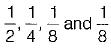respectively.

The entropy of the system is

Solution:

The entropy of the system is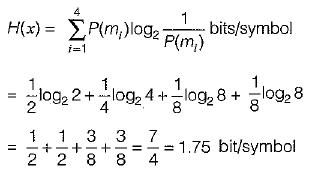QUESTION: 10

A communication channel with AWGN has a BW of 4 kHz and an SNR pf 15. Its channel capacity is

Solution:

Channel capacity is